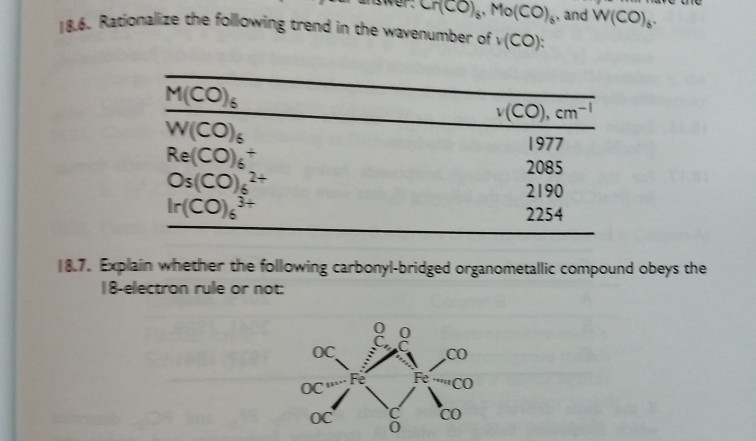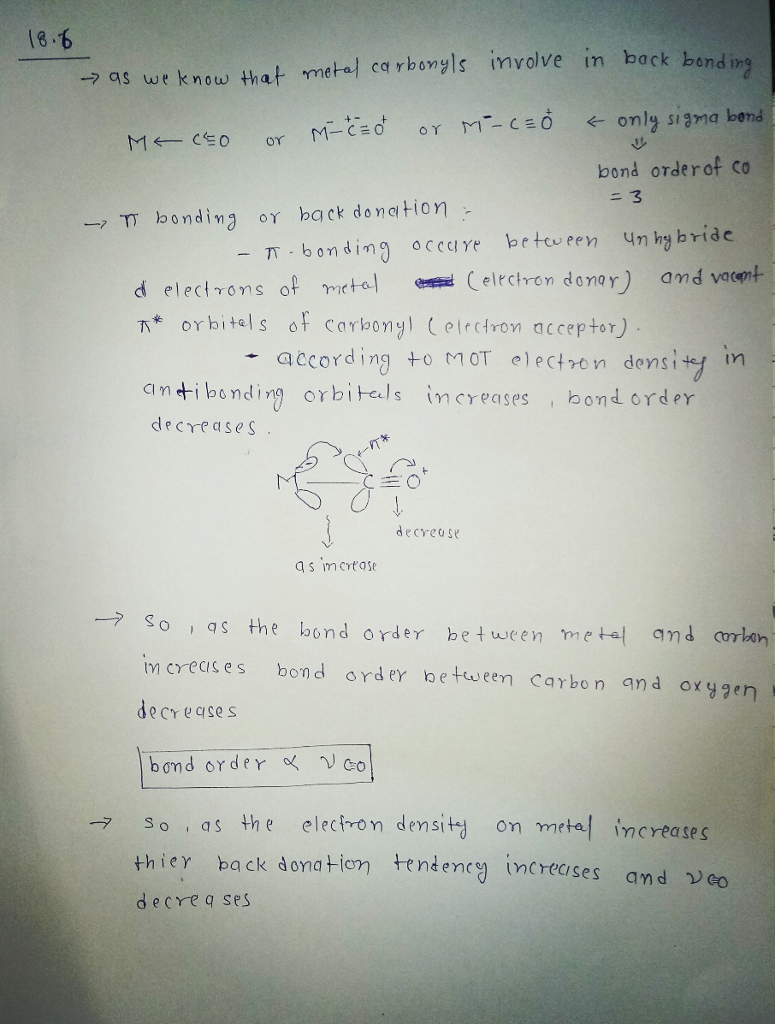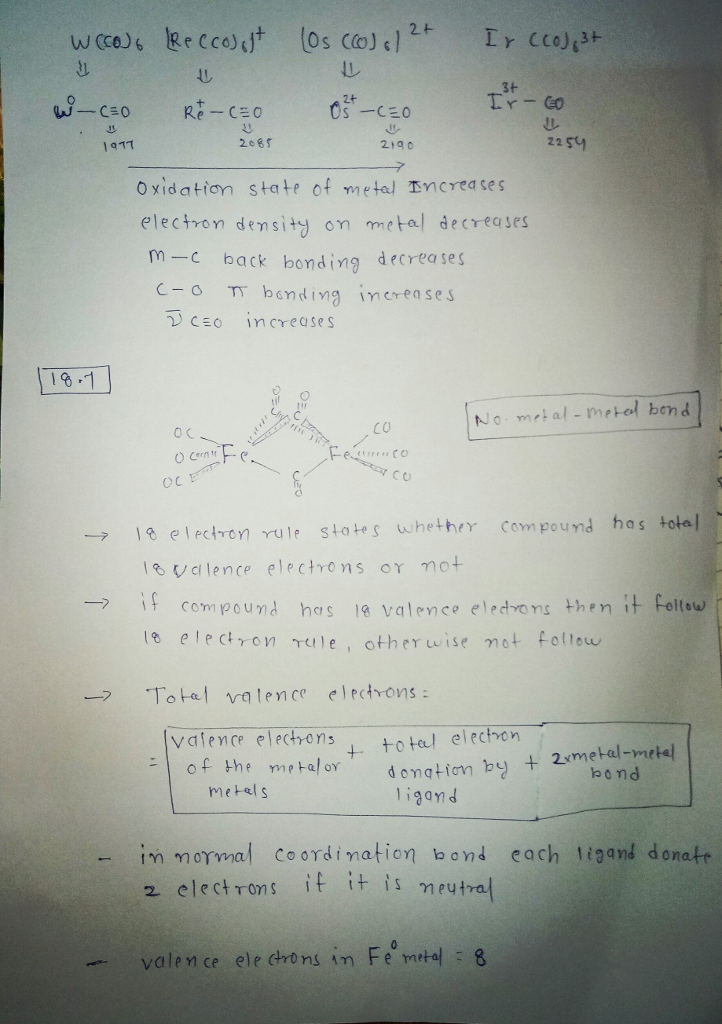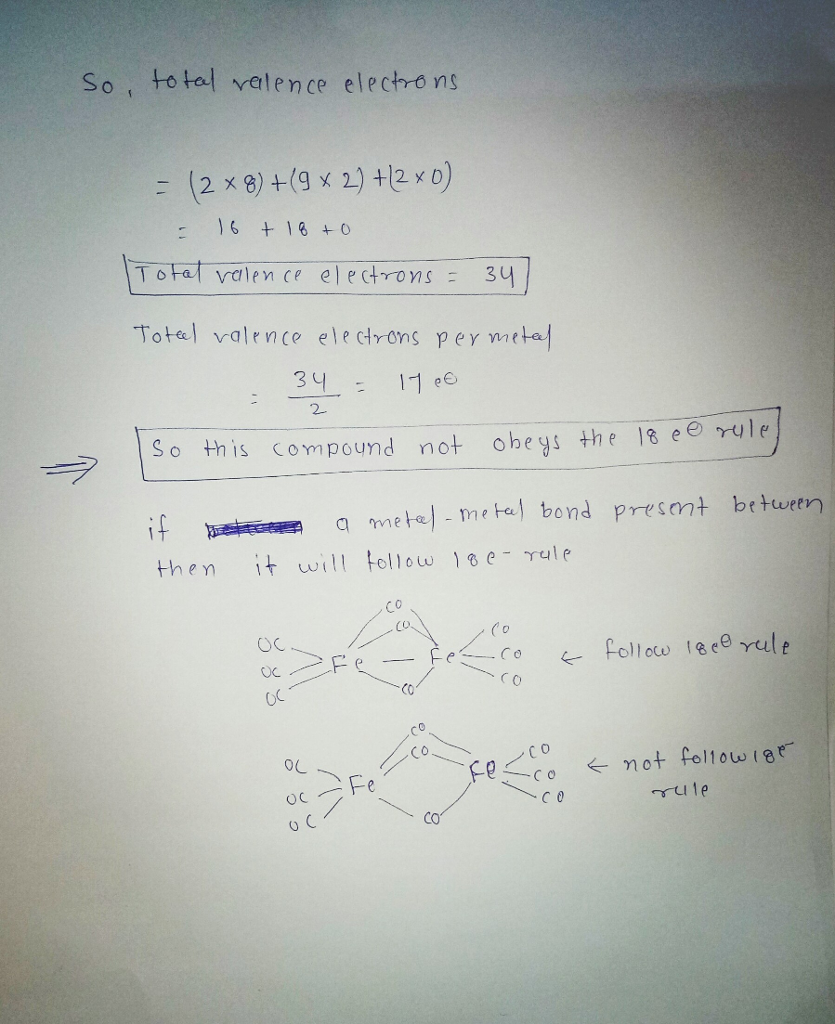# please explain each step for both problems twe CHcoMo(CO) and W(CO) alize the following trend in...please explain each step for both problems

twe CHcoMo(CO) and W(CO) alize the following trend in the wavenumber of (CO) M(CO) Re(CO)s Os(CO)s Ir(CO)s v (CO), cm-1 1977 2085 2190 2254 2- 187. Explain whether the following carbonyl-bridged organometallic compound obeys the 18-electron rule or not co FeCo OC Fe OC OC 0#### Earn Coin

Coins can be redeemed for fabulous gifts.

Similar Homework Help Questions
• ### 5. (a) When [Mo(?5 -C5H5)(CO)3]2 is heated, it loses two CO ligands to become [Mo(?5 -C5H5)(CO)2]2,...

5. (a) When [Mo(?5 -C5H5)(CO)3]2 is heated, it loses two CO ligands to become [Mo(?5 -C5H5)(CO)2]2, and the energies of the CO stretches change from 1960 cm?1 and 1915 cm?1 to 1859 cm?1 and 1819 cm?1 . The Mo-Mo bond length also shortens by 80 pm. Suggest plausible chemical structures for each of the compounds, and explain the change in energy of the CO stretches. (b) Explain the following trend in the energy of the CO stretch. Predict the trend...

• ### 2. For each of the following compounds, 1) write the formal oxidation state of the transition...

2. For each of the following compounds, 1) write the formal oxidation state of the transition metal and 2) the corresponding number of d electrons. 3) State whether or not each one is likely to be stable enough to be characterized. (a) [Re(CO)5] (b) [Cr(η6 -C6H6)2] (c) [Mo(CO)3(PPh3)3] (d) [Fe(η2 -C2H4)(CO)4] (e) [Zr(η5 -C5H5)Cl(OH)] (f) [Co(η5 -C5H5)2] 3. Identify the first-row transition metal (M) that follows the 18-electron rule for each of the following compounds (show how you arrived at...

• ### 2. For each of the following compounds, 1) write the formal oxidation state of the transition met...

2. For each of the following compounds, 1) write the formal oxidation state of the transition metal and 2) the corresponding number of d electrons. 3) State whether or not each one is likely to be stable enough to be characterized. (a) [Re(CO)5] (b) [Cr(η6 -C6H6)2] (c) [Mo(CO)3(PPh3)3] (d) [Fe(η2 -C2H4)(CO)4] (e) [Zr(η5 -C5H5)Cl(OH)] (f) [Co(η5 -C5H5)2] 3. Identify the first-row transition metal (M) that follows the 18-electron rule for each of the following compounds (show how you arrived at...

Free Homework App